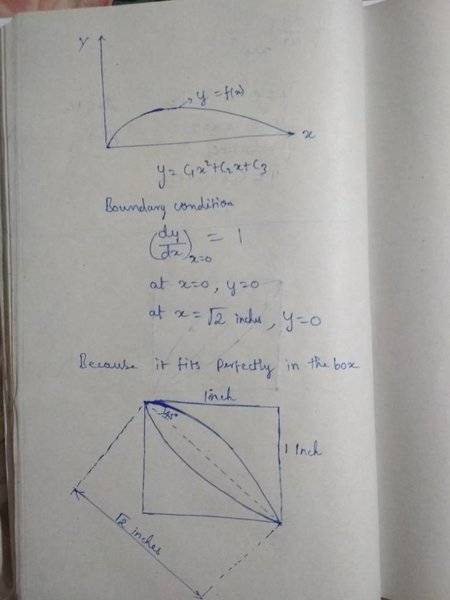# Area of Airfoil cross-section

New poster has been reminded to post schoolwork in the Homework Help forums and use the Template
Not really sure if this thread belongs here but im supposed to figure out the radius of gyration, section modulus, and Q values at the neutral axis for this symmetric wing cross section. The only problem is that im not even really sure how to find the area of the cross section. i know that it'll be ∫(f(x)-g(x)) dx from 0-1 (pretty much the only hint that we are given is that the cross section can "fit perfectly in a 1in x 1in box) but how i even find f(x) and g(x) is beyond me.

#### Attachments

•Screen Shot 2018-07-23 at 12.08.23 AM.png
12 KB · Views: 1,386

Poster has been reminded to watch for misplaced homework threads, and not to do the student's homework for them.
There is a high chance for my answer to be wrong.If its right, by solving the equatuon with the boundary condition will give you the curve(refer the image).
If X axis in the diagram is your neutral axis then it is better to find x as a function of y by using the same boundary condition.(To calculate 2nd moment of area about the x axis).#### Attachments

Last edited:
Klystron
Gold Member
Alec, for future aerodynamic calculations consider determining the mean aerodynamic chord (MAC) of the wing section under study.
https://en.wikipedia.org/wiki/Chord_(aeronautics)

Before digital computers became common, aircraft engineers adopted many terms and techniques from boat building including chords.